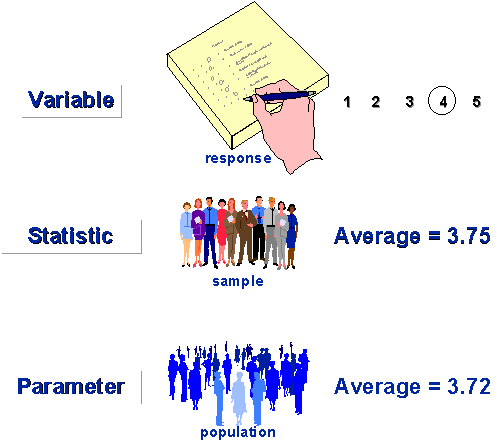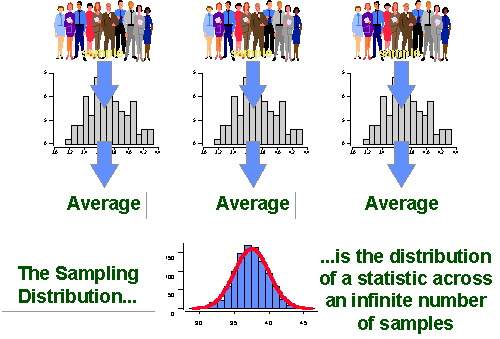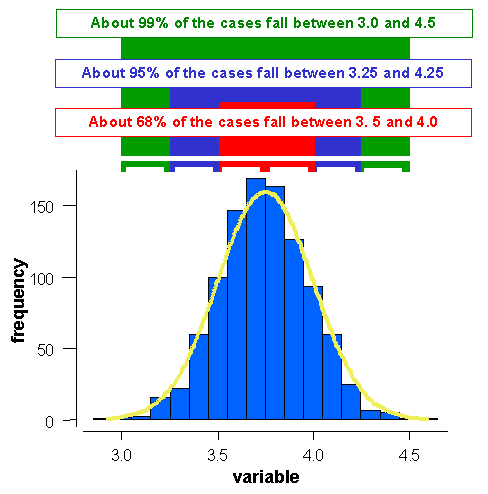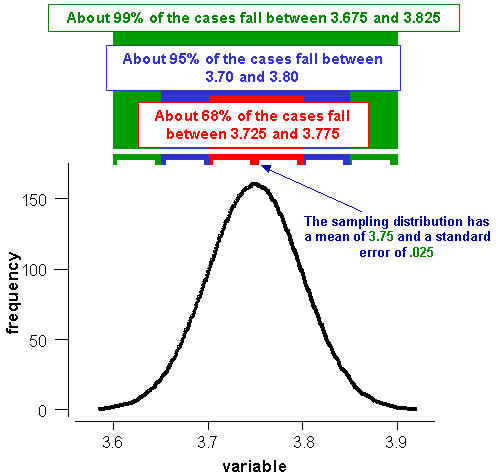Statistical Terms in Sampling

# Statistical Terms in Sampling

Let’s begin by defining some very simple terms that are relevant here. First, let’s look at the results of our sampling efforts. When we sample, the units that we sample – usually people – supply us with one or more responses. In this sense, a response is a specific measurement value that a sampling unit supplies. In the figure, the person is responding to a survey instrument and gives a response of `4`. When we look across the responses that we get for our entire sample, we use a statistic. There are a wide variety of statistics we can use – mean, median, mode, and so on. In this example, we see that the mean or average for the sample is `3.75`. But the reason we sample is so that we might get an estimate for the population we sampled from. If we could, we would much prefer to measure the entire population. If you measure the entire population and calculate a value like a mean or average, we don’t refer to this as a statistic, we call it a parameter of the population.## The Sampling Distribution

So how do we get from our sample statistic to an estimate of the population parameter? A crucial midway concept you need to understand is the sampling distribution. In order to understand it, you have to be able and willing to do a thought experiment. Imagine that instead of just taking a single sample like we do in a typical study, you took three independent samples of the same population. And furthermore, imagine that for each of your three samples, you collected a single response and computed a single statistic, say, the mean of the response. Even though all three samples came from the same population, you wouldn’t expect to get the exact same statistic from each. They would differ slightly just due to the random “luck of the draw” or to the natural fluctuations or vagaries of drawing a sample. But you would expect that all three samples would yield a similar statistical estimate because they were drawn from the same population. Now, for the leap of imagination! Imagine that you did an infinite number of samples from the same population and computed the average for each one. If you plotted them on a histogram or bar graph you should find that most of them converge on the same central value and that you get fewer and fewer samples that have averages farther away up or down from that central value. In other words, the bar graph would be well described by the bell curve shape that is an indication of a “normal” distribution in statistics. The distribution of an infinite number of samples of the same size as the sample in your study is known as the sampling distribution.We don’t ever actually construct a sampling distribution. Why not? You’re not paying attention! Because to construct it we would have to take an infinite number of samples and at least the last time I checked, on this planet infinite is not a number we know how to reach. So why do we even talk about a sampling distribution? Now that’s a good question! Because we need to realize that our sample is just one of a potentially infinite number of samples that we could have taken. When we keep the sampling distribution in mind, we realize that while the statistic we got from our sample is probably near the center of the sampling distribution (because most of the samples would be there) we could have gotten one of the extreme samples just by the luck of the draw. If we take the average of the sampling distribution – the average of the averages of an infinite number of samples – we would be much closer to the true population average – the parameter of interest. So the average of the sampling distribution is essentially equivalent to the parameter. But what is the standard deviation of the sampling distribution (OK, never had statistics? There are any number of places on the web where you can learn about them or even just brush up if you’ve gotten rusty. This isn’t one of them. I’m going to assume that you at least know what a standard deviation is, or that you’re capable of finding out relatively quickly). The standard deviation of the sampling distribution tells us something about how different samples would be distributed. In statistics it is referred to as the standard error (so we can keep it separate in our minds from standard deviations. Getting confused? Go get a cup of coffee and come back in ten minutes…OK, let’s try once more… A standard deviation is the spread of the scores around the average in a single sample. The standard error is the spread of the averages around the average of averages in a sampling distribution. Got it?)

## Sampling Error

In sampling contexts, the standard error is called sampling error. Sampling error gives us some idea of the precision of our statistical estimate. A low sampling error means that we had relatively less variability or range in the sampling distribution. But here we go again – we never actually see the sampling distribution! So how do we calculate sampling error? We base our calculation on the standard deviation of our sample. The greater the sample standard deviation, the greater the standard error (and the sampling error). The standard error is also related to the sample size. The greater your sample size, the smaller the standard error. Why? Because the greater the sample size, the closer your sample is to the actual population itself. If you take a sample that consists of the entire population you actually have no sampling error because you don’t have a sample, you have the entire population. In that case, the mean you estimate is the parameter.## The 68, 95, 99 Percent Rule

You’ve probably heard this one before, but it’s so important that it’s always worth repeating… There is a general rule that applies whenever we have a normal or bell-shaped distribution. Start with the average – the center of the distribution. If you go up and down (i.e., left and right) `one` standard unit, you will include approximately `68%` of the cases in the distribution (i.e., `68%` of the area under the curve). If you go up and down `two` standard units, you will include approximately `95%` of the cases. And if you go plus-and-minus `three` standard units, you will include about `99%` of the cases. Notice that I didn’t specify in the previous few sentences whether I was talking about standard deviation units or standard error units. That’s because the same rule holds for both types of distributions (i.e., the raw data and sampling distributions). For instance, in the figure, the mean of the distribution is `3.75` and the standard unit is `.25` (If this was a distribution of raw data, we would be talking in standard deviation units. If it’s a sampling distribution, we’d be talking in standard error units). If we go up and down one standard unit from the mean, we would be going up and down `.25` from the mean of `3.75`. Within this range – `3.5 to 4.0` – we would expect to see approximately `68%` of the cases. This section is marked in red on the figure. I leave to you to figure out the other ranges. But what does this all mean you ask? If we are dealing with raw data and we know the mean and standard deviation of a sample, we can predict the intervals within which `68, 95 and 99%` of our cases would be expected to fall. We call these intervals the – guess what – `68, 95 and 99%` confidence intervals.Now, here’s where everything should come together in one great aha! experience if you’ve been following along. If we had a sampling distribution, we would be able to predict the `68, 95 and 99%` confidence intervals for where the population parameter should be! And isn’t that why we sampled in the first place? So that we could predict where the population is on that variable? There’s only one hitch. We don’t actually have the sampling distribution (now this is the third time I’ve said this in this essay)! But we do have the distribution for the sample itself. And we can from that distribution estimate the standard error (the sampling error) because it is based on the standard deviation and we have that. And, of course, we don’t actually know the population parameter value – we’re trying to find that out – but we can use our best estimate for that – the sample statistic. Now, if we have the mean of the sampling distribution (or set it to the mean from our sample) and we have an estimate of the standard error (we calculate that from our sample) then we have the two key ingredients that we need for our sampling distribution in order to estimate confidence intervals for the population parameter.

Perhaps an example will help. Let’s assume we did a study and drew a single sample from the population. Furthermore, let’s assume that the average for the sample was `3.75` and the standard deviation was `.25`. This is the raw data distribution depicted above. now, what would the sampling distribution be in this case? Well, we don’t actually construct it (because we would need to take an infinite number of samples) but we can estimate it. For starters, we assume that the mean of the sampling distribution is the mean of the sample, which is `3.75`. Then, we calculate the standard error. To do this, we use the standard deviation for our sample and the sample size (in this case N=`100`) and we come up with a standard error of `.025` (just trust me on this). Now we have everything we need to estimate a confidence interval for the population parameter. We would estimate that the probability is `68%` that the true parameter value falls between `3.725 and 3.775` (i.e., `3.75` plus and minus `.025`); that the `95%` confidence interval is `3.700 to 3.800`; and that we can say with `99%` confidence that the population value is between `3.675 and 3.825`. The real value (in this fictitious example) was `3.72` and so we have correctly estimated that value with our sample.